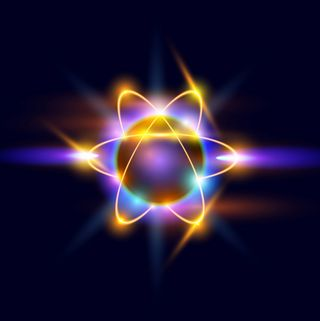# Wacky Physics: New Uncertainty About the Uncertainty PrincipleOne of the most often quoted, yet least understood, tenets of physics is the uncertainty principle.

Formulated by German physicist Werner Heisenberg in 1927, the rule states that the more precisely you measure a particle's position, the less precisely you will be able to determine its momentum, and vice versa.

The principle is often invoked outside the realm of physics to describe how the act of observing something changes the thing being observed, or to point out that there's a limit to how well we can ever really understand the universe.

While the subtleties of the uncertainty principle are often lost on nonphysicists, it turns out the idea is frequently misunderstood by experts, too. But a recent experiment shed new light on the maxim and led to a novel formula describing how the uncertainty principle really works.

Perplexing logic

The uncertainty principle only applies in the quantum mechanical realm of the very small, on scales of subatomic particles. Its logic is perplexing to the human mind, which is acclimated to the macroscopic world, where measurements are only limited by the quality of our instruments.

But in the microscopic world, there truly is a limit to how much information we can ever glean about an object.

For example, if you make a measurement to find out exactly where an electron is, you will only be able to get a hazy idea of how fast it's moving. Or you might choose to determine an electron's momentum fairly precisely, but then you will have only a vague idea of its location.  [Graphic: Nature's Tiniest Particles Explained]

Heisenberg originally explained the limitation using a thought experiment. Imagine shining light at a moving electron. When a photon, or particle of light, hits the electron, it will bounce back and record its position, yet in the process of doing so, it has given the electron a kick, thereby changing its speed.

The wavelength of the light determines how precisely the measurement can be made. The smallest wavelength of light, called gamma-ray light, can make the most precise measurements, but it also carries the most energy, so an impacting gamma-ray photon will deliver a stronger kick to the electron, thereby disturbing its momentum the most.

Though not imparting as much disruption to the electron's momentum, a longer wavelength of light wouldn't allow as precise a measurement.

Marbles and billiard balls

"In the early days of quantum mechanics, people interpreted the uncertainty relation in terms of such back-reactions of the measurement process," said physicist Georg Sulyok of the Institute of Atomic and Subatomic Physics in Austria. "But this explanation is not 100 percent correct."

Sulyok worked with a research team, led by physicists Masanao Ozawa of Japan's Nagoya University and Yuji Hasegawa of Vienna University of Technology in Austria, to calculate and experimentally demonstrate how much of the uncertainty principle is due to the effects of measurement, and how much is simply due to the basic quantum uncertainty of all particles.

In quantum mechanics, particles can't be thought of as marbles or billiard balls — tiny, physically distinct objects that travel along a straight course from point A to point B. Instead, particles can behave like waves, and can only be described in terms of the probability that they are at point A or point B or somewhere in between.

This is also true of a particle's other properties, such as its momentum, energy and spin.

This probabilistic nature of particles means there will always be imprecision in any quantum measurement, no matter how little that measurement disturbs the system it is measuring.

"This has nothing to do with error or disturbances due to a measurement process, but is a basic fundamental property that every quantum mechanical particle has," Sulyok told LiveScience. "In order to describe the basic uncertainty together with measurement errors and disturbances, both particle and measurement device in a successive measurement have to be treated in the framework of quantum theory."

Calculating the uncertainty

To test how much this fundamental property contributes to the overall uncertainty, the researchers devised an experimental setup to measure the spin of a neutron in two perpendicular directions. These quantities are related, just as position and momentum are, so that the more precise a measurement is made of one, the less precise a measurement can be made of the other.

The physicists used magnetic fields to manipulate and measure the neutrons' spin, and conducted a series of measurements where they systematically changed the parameters of the measuring device.

"You have this basic uncertainty, and then by measuring you add an additional uncertainty," Sulyok said. "But with an apparatus performing two successive measurements, you can identify the different contributions."

Using their data, the physicists were able to calculate just how the different types of uncertainty add together and influence each other. Their new formula doesn't change the conclusion of the Heisenberg uncertainty principle, but it does tweak the reasoning behind it.

"The explanation that Heisenberg gave is very intuitive," Sulyok said. "On a popular science level it is hardly ever distinguished at all, and sometimes it's even not correctly explained in university textbooks. The quantum-mechanically correct calculation reinforced by our experimental data is a valuable step in achieving a more consistent view on the uncertainty principle."

The results of the study were published in January 2012 in the journal Nature Physics.